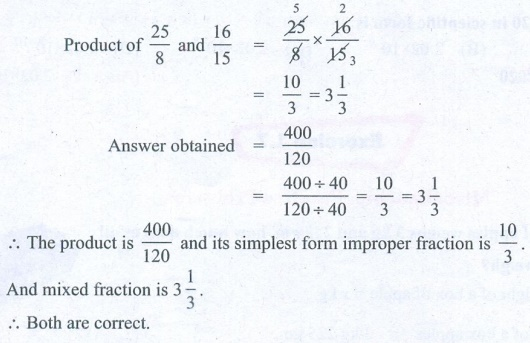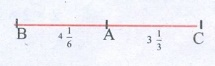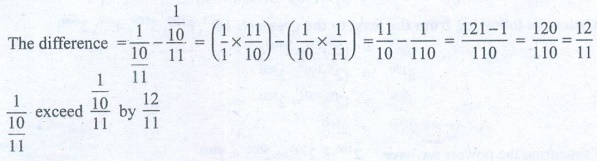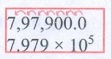Home | | Maths 8th Std | Exercise 1.7

# Exercise 1.7

8th Maths : Chapter 1 : Numbers: Exercise 1.7: Numerical Example solved problems and Text book back Numerical problems Questions with Solution

Exercise 1.7

Miscellaneous Practice Problems

1. If 3/4 of a box of apples weighs 3 kg and 225 gm, how much does a full box of apples weigh?Solution:

Let the total weight of a box of apple = x kg.

Weight of 3/4 of a box apples = 3 kg 225 gm.

= 3.225 kg

(3/4) × x = 3225

x = { [3.225 × 4] / 3 } kg = [1.075 × 4 ] kg = 4.3 kg

= 4 kg 300 gm.

Weight of the box of apples = 4 kg 300 gm.

2. Mangalam buys a water jug of capacity 3 (4/5) litre. If she buys another jug which is 2 (2/3) times as large as the smaller jug, how many litre can the larger one hold?

Solution:

Capacity of the small water jug = 3 4/5 litres.

Capacity of the big jug = 2 2/3 times the small one.

= 2(2/3) × 3(4/5) = [8/3] × [19/5] = 152/15 == 10 (2/15) litres

Capacity of the large jug = 10 2/15 litres =litres.

3. Ravi multiplied 25/8 and 16/15 and he says that the simplest form of this product is 10/3 and Chandru says the answer in the simplest form is 3 1/3 Who is correct? (or) Are they both correct? Explain.

Solution:Product of 25/8 and 16/15 = [25/8] × [16/15]

= 10/3 = 3 (1/3)

= [400 ÷ 40] / [120 ÷ 40] = 10/3 = 3 (1/3)

The product is 400/120 and its simplest form improper fraction is 10/3.

And mixed fraction is 3 (1/3).

Both are correct.

4. Find the length of a room whose area is 153/10 sq.m and whose breadth is 2 11/20 m.

Solution:Length of the room × Breadth = Area of the room

Breadth of the room = 2 (11/20) m

Area of the room = 153/10 sq.m

Length × 2(11/20) = 153/10

Length = [153/10] ÷ [2 (11/20)] = 153/10 ÷ 51/20 = 153/10 × 20/51 = 6 m

Length of the room = 6 m

5. There is a large square portrait of a leader that covers an area of 4489 cm2. If each side has a 2 cm liner, what would be its area?

Solution:Area of the square  = 4489 cm2

(side)2 = 4489 cm2

(side)2 = 67 × 67

side2 = 672

Length of a side = 67

Length of a side with liner = 67 + 2 + 2 cm

= 71 cm

Area of the larger square = 71 × 71 cm2

= 5041 cm2

Area of the liner = Area of big square − Area of small square

= (5041 − 4489) cm2

= 552 cm2

6. A greeting card has an area 90 cm2. Between what two whole numbers is the length of its side?

Solution:

Area of the greeting card = 90 cm2

(side)2 = 90 cm2(side)2 = 2 × 5 × 3 × 3 = 2 × 5 × 32

√(side)2 = √(2×5×32)

side = 3 √(2×5)

side = 3 √10 cm

side = 3 × 3.2 cm

side = 9.6 cm

Side lies between the whole numbers 9 and 10.

7. 225 square shaped mosaic tiles, each of area 1 square decimetre exactly cover a square shaped verandah. How long is each side of the square shaped verandah?

Solution:

Area of one tile = 1 sq.decimeter

Area of 225 tiles = 225 sq.decimeter

225 square tiles exactly covers the square shaped verandah.

Area of 225 tiles = Area of the verandah

Area of the verandah = 225 sq.decimeter

side × side = 15 × 15 sq.decimeter

side = 15 decimeters

Length of each side of verandah = 15 decimeters.

8. If 3√1906624 × √x = 3100, find x.

Solution:9. If 2m-1 + 2m+1 = 640, then find m.

Solution:

Given 2m−1 + 2m+1 = 640

2m−1 + 2m+1 = 128 + 512  [consecutive powers of 2]

2m−1 + 2m+1 = 27 + 29

m – 1 = 7

m = 7 + 1

m = 8

Powers of 2

2,4,8,16,32,64,128,256,512,…

10. Give the answer in scientific notation:

A human heart beats at an average of 80 beats per minute. How many times does it beat in i) an hour? ii) a day? iii) a year? iv) 100 years?

Solution:

Heart beat per minute = 80 beats

(i) an hour

One hour = 60 minutes

Heart beat in an hour = 60 × 80

= 4800 = 4.8 × 103

(ii) In a day

One day = 24 hours = 24 × 60 minutes

Heart beat in one day = 24 × 60 × 80 = 24 × 4800 = 115200

= 1.152 × 105

(iii) a year

One year = 365 days = 365 × 24 hours = 365 × 24 × 60 minutes

Heart beats in a year = 365 × 24 × 60 × 80

= 42048000 = 4.2048 × 107

(iv) 100 years

Heart beats in one year = 4.2048 × 107

Heart beats in 100 years = 4.2048 × 107 × 100 = 4.2048 × 107 × 102

= 4.2048 × 109

Challenging Problems

11. In a map, if 1 inch refers to 120 km, then find the distance between two cities B and C which are 4(1/6) inches and 3(1/3) inches from the city A which lies between the cities B and C.

Solution:1 inch =120 km

Distance between A and B = 4 (1/6) inches

Distance between A and C = 3 (1/3) inches

Distance between B and C = 4 (1/6) + 3 (1/3) inches

= 25/6 + 10/3 = 25/6 + 20/6 = [25 + 20] / 6 = 45/6 inches

1 inch = 120 km

45/6 inches = [45/6] × 120 km = 900 km

Distance between B and C = 900 km

12. Give an example and verify each of the following statements

(i) The collection of all non-zero rational numbers is closed under division.

(ii) Subtraction is not commutative for rational numbers.

(iii) Division is not associative for rational numbers.

(iv) Distributive property of multiplication over subtraction is true for rational numbers. That is, a (b c ) = ab ac .

(v) The mean of two rational numbers is rational and lies between them

Solution:(i) Let a = 5/6 and b = −4/3 be two non zero rational numbers.

a ÷ b = 5/6 ÷  −4/3 =  5/6 × 3/−4 = 5/−8 is in Q

Collection of non−zero rational numbers are closed under division.

(ii) Let a = 1/2 and b = −5/6 be two rational numbers.

ab = 1/2  − (−5/6) = 1/2 + (+5/6) = 3/6 + 5/6 = [3 + 5] / 6 = 8/6

= 1 (2/6) = 1 1/3

b – a = −5/6 – 1/2 = −5/6 – 3/6 = [−5 −3] / 6 = −8/6 = −1 (1/3)

a − b ≠ b − a

Subtraction is not commutative for rational numbers.

(iii) Let a = 2/5, b = 6/5,  c =  3/5  be three rational numbers.

a ÷ (b ÷ c) = 2/5 ÷ (6/5 ÷ 3/5) = 2/5 ÷  (6/5 × 5/3)

= 2/5 ÷ 2/1 = 2/5 × 1/2 = 1/5 ……….(1)

(a ÷ b) ÷ c = (2/5 ÷ 6/5) ÷ 3/5

= (2/5 × 5/6) ÷ 3/5 = 1/3 ÷ 3/5 = 1/3 × 5/3 = 5/9 ……..(2)

From (1) and (2) 1/5  ≠  5/9

a ÷ (b ÷ c) ≠ (a ÷ b) ÷ c

Division is not associative for rational numbers.

(iv) Let a = 2/9, b = 3/6 , c = 1/3 be three rational numbers

To prove a × (bc) = abac

a × (b − c) = 2/9 × (3/6 − 1/3)

= [2/9] × ( [3 − (1 × 2)] / 6 ) = [2/9] × [(3 – 2) / 6]

= 2/9 × 1/6 = 1 / 27 ……..(1)

abac = (2/9 × 3/6) – (2/9 × 1/3) = 1/9 – 2/27

= [(1 × 3) – 2] / 27 = [3 – 2] / 27 = 1/27  ….(2)

From (1) and (2)

a ×  (bc) = abac.

Distributivity of multiplication over subtraction is true for rational numbers.

(v) Let a = 2/11 and b = 5/6 be two rational numbers.

Mean of a and b is c =1/2  (a + b) = 1/2 (2/11 + 5/6) = 1/2 ((2×6) + (5×11) / 66)

= 1/2 × ([12 + 55] / 66) = 1/2 × 67/66 = 67/132 is in Q.

Also 2/11 = [2 × 12] / [11 × 12] = 24/132

5/6 = [5 × 22] / [6 × 22] = 110 / 132

24/132 < 67/132 < 110/132

The mean lies between the given rational numbers 2/11 and 5/6.

13. If 1/4 of a ragi adai weighs 120 grams, what will be the weight of 2/3 of the same ragi adai?

Solution:

Let the weight of 1 ragi adai = x grams

given 1/4 of x = 120 gm

(1/4) × x = 120

x = 120 × 4

x = 480 gm

2/3 of the adai = 2/3  × 480 gm = 2 × 160 gm = 320 gm

2/3 of the weight of adai = 320 gm

14. If p + 2q = 18 and pq = 40 , find 2/p + 1/q

Solution:

Given: p + 2q = 18 ….(1)

pq = 40  ….(2)

2/p + 1/q = [(2 × q) + (l × p)] / pq = [2q + p ] / pq = 18/40 [ from (1) and (2)]

2/p + 1/q = 9/20

15. Find x if 5(x/5) × 3(3/4) = 21.

Solution:[ 5 x/5 ] × [3 3/4] = 21

[(25 + x) / 5] × [15/4] = 21

[ 25+ x ] / 5 = 21 ÷ [15/4]

[25+ x ] / 5 = 21 × [4/15]

[25+ x ] / 5 = 28/5

25 + x = [28 × 5] / 5

25 + x = 28

x = 28 – 25

x = 3

16. By how much does 1/(10/11) exceed (1/10) / 11 ?

Solution:The difference = { 1 / [10/11] } – { [1/10] / 11 } = (1/1 × 11/10) – (1/10 × 1/11) = 11/10 – 1/110 = [121 – 1] / 110 = 120/110 = 12/11

{ 1 / [10/11] } exceed  { [1/10] / 11 } by 12/11

17. A group of 1536 cadets wanted to have a parade forming a square design. Is it possible? If it is not possible, how many more cadets would be required?

Solution:

Number of cadets to form square design

1536 = 2 × 2 × 2 × 2 × 2 × 2 × 2 × 2 × 2 × 3

The numbers 2 and 3 are unpaired

It is impossible to have the parade forming square design with 1536 cadets.39 × 39 = 1521

Also 40 × 40 = 1600

We have to add (1600 − 1536) = 64 to make 1536 a perfect square.

64 more cadets would be required to form the square design.

18. Evaluate: √286225 and use it to compute √2862.25 + √28.6225

Solution:√286225 = 535

√2862.25 = √[286225 / 100] = √286225 / √100 = 535/10 = 53.5

√28.6225 = √[286225 / 1000] =  √286225/√10000 = 535/100 =  5.35

√2862.25 + √28.6225 = 53.5 + 5.35 = 58.85

19. Simplify: (3.769 × 105) + (4.21 × 105)

Solution:(3.769 × 105) + (4.21 × 105) = 3,76,900 + 4,21,000

= 7,97,900 = 7.979 × 105

20. Order the following from the least to the greatest: 1625 , 8100 , 3500 , 4400 , 2600

Solution:

1625 = (24)25 = 2100

8100 =  (23)100 = 2300

4400 = (22)400 = 2800

2600 =  2600

Comparing the powers we have. 2100 < 2300 < 2600 < 2800

The required order : 1625 , 8100, 2600, 3500, 4400

Exercise 1.7

Miscellaneous Practice Problems

1. 4 kg 300 gm

2. 10 2/5 litre

3. both are correct

4. 6 m

5. 552 cm2

6. 9 cm and 10 cm

7. 15 decimetre

8. 625

9. 8

10. (i) 4.8 × 103 (ii) 1.152 × 105 (iii) 4.2048 × 107 (iv) 4.2048 × 109

Challenging Problems

11. 900 km

13. 320 gm

14. 9/20

15. x = 3

16. 12/11

17. No, 64

18. 58.85

19. 7.979 × 105

20. 8100, 2600, 3500, 4400, 1625

Tags : Questions with Answers, Solution | Numbers | Chapter 1 | 8th Maths , 8th Maths : Chapter 1 : Numbers
Study Material, Lecturing Notes, Assignment, Reference, Wiki description explanation, brief detail
8th Maths : Chapter 1 : Numbers : Exercise 1.7 | Questions with Answers, Solution | Numbers | Chapter 1 | 8th Maths